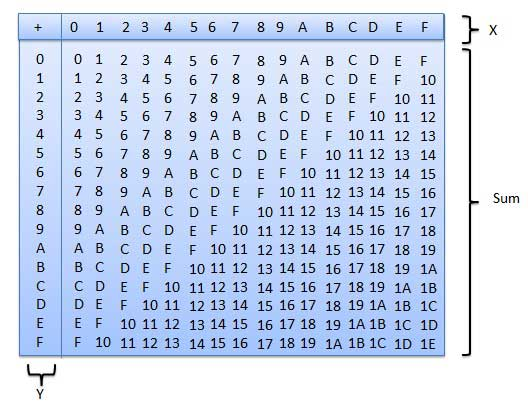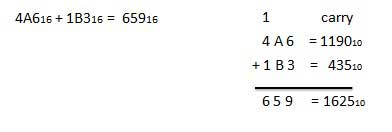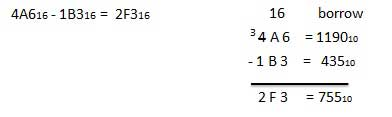# Hexadecimal Arithmetic

Advertisements

## Hexadecimal Number System

Following are the characteristics of a hexadecimal number system.

• Uses 10 digits and 6 letters, 0,1,2,3,4,5,6,7,8,9,A,B,C,D,E,F.

• Letters represents numbers starting from 10. A = 10, B = 11, C = 12, D = 13, E = 14, F = 15.

• Also called base 16 number system.

• Each position in a hexadecimal number represents a 0 power of the base (16). Example − 160

• Last position in a hexadecimal number represents an x power of the base (16). Example − 16x where x represents the last position - 1.

### Example

Hexadecimal Number − 19FDE16

Calculating Decimal Equivalent −

Step Hexadecimal Number Decimal Number
Step 1 19FDE16 ((1 × 164) + (9 × 163) + (F × 162) + (D × 161) + (E × 160))10
Step 2 19FDE16 ((1 × 164) + (9 × 163) + (15 × 162) + (13 × 161) + (14 × 160))10
Step 3 19FDE16 (65536 + 36864 + 3840 + 208 + 14)10
Step 4 19FDE16 10646210

Note − 19FDE16 is normally written as 19FDE.

## Hexadecimal Addition

Following hexadecimal addition table will help you greatly to handle Hexadecimal addition.To use this table, simply follow the directions used in this example − Add A16 and 516. Locate A in the X column then locate the 5 in the Y column. The point in 'sum' area where these two columns intersect is the sum of two numbers.

```A16 + 516 = F16.
```

### Example − Addition## Hexadecimal Subtraction

The subtraction of hexadecimal numbers follow the same rules as the subtraction of numbers in any other number system. The only variation is in borrowed number. In the decimal system, you borrow a group of 1010. In the binary system, you borrow a group of 210. In the hexadecimal system you borrow a group of 1610.

### Example - SubtractionAdvertisements